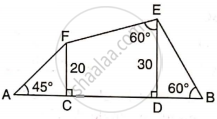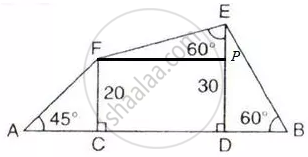# Find Ab. - Mathematics

Sum

Find AB.#### Solution

Consider the figureFrom right triangle ACF

tan 45° = (20)/"AC"

1 = (20)/"AC"

AC = 20 cm

From triangle DEB

tan 60° = (30)/"BD"

sqrt(3) = (30)/"BD"

BD = (30)/sqrt(3) = 17.32 cm

Given FC = 20, ED = 30, So EP = 10 cm
Therefore

tan 60° = "FP"/"EP"

sqrt(3)= "FP"/(10)

FP = 10sqrt(3) = 17.32 cm

Thus AB = AC + CD + BD = 54.64 cm.

Concept: Solution of Right Triangles
Is there an error in this question or solution?

#### APPEARS IN

Selina Concise Mathematics Class 9 ICSE
Chapter 24 Solution of Right Triangles [Simple 2-D Problems Involving One Right-angled Triangle]
Exercise 24 | Q 7 | Page 303